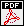• My work is supported by NSF grants DMS 1358648 (1200898, 2012-2016), DMS 1600782 (2016-2019), and DMS 1752345 (CAREER, 2018-2023).

• Before coming to OSU I was trained at UPenn ('10-'12) as a postdoc. I also spent some time at Yale (as a research associate, '12-'13) and at IAS (as a von Neumann fellow, '15-'16).

• I finished my Ph.D. at Rutgers in 2010 under the guidance by prof. Van Vu; cv.

• Co-authors: Scott Aaronson, Jozsef Balogh, Mei-Chu Chang, Yen Do, Sean Meehan, Oanh Nguyen, Sean O'Rourke, Elliot Paquette, Endre Szemeredi, Terence Tao, Van Vu, Melanie Matchett Wood.

Anti-concentration of random walks

 Anti-concentration of inhommogeneous random walks in non-abelian groups (preprint).Small probability, inverse theorems, and applications (with Van Vu, on the occasion of Paul Erdos' 100th anniversary, Bolyai Society Mathematical Studies, Vol. 25 (2013)).Inverse Littlewood-Offord problems and the singularity of random symmetric matrices (Duke Mathematics Journal Vol. 161, 4 (2012), 545-586).On the least singular value of random symmetric matrices (Electronic Journal of Probability 17 (2012), no. 53).Optimal inverse Littlewood-Offord theorems (with Van Vu, Advances in Mathematics, Vol. 226 6 (2011), 5298-5319) (Erratum).Universality phenomenon in random matrices

 Random matrices over finite fields: methods and results (with Kyle Luh and Sean Meehan, in preparation).Cokernels of adjacency matrices of random r-regular graphs (with Melanie Matchett Wood, submitted).Random integral matrices: universality of surjectivity and the cokernel (with Melanie Matchett Wood, submitted).Random matrices: overcrowding estimates for the spectrum (Journal of Functional Analysis, (2018) Volume 275, Issue 8, 2197-2224).Normal vector of a random hyperplane (with Van Vu, International Mathematics Research Notices, (2018) Issue 6, 1754-1778).Random matrices: tail bounds for gaps between eigenvalues (with Terence Tao and Van Vu, Probability Theory and Related Fields, (2017), Vol. 167, 3, 777-816).The elliptic law (with Sean O'Rourke, International Mathematics Research Notices (2015), Vol. 2015, 7620-7689).On the concentration of random multilinear forms and the universality of random block matrices (with Sean O'Rourke, Probability Theory and Related Fields (2015), Vol. 162, 1, 97-154).Random doubly stochastic matrices: the circular law (Annals of Probability (2014), Vol. 42, No. 3, 1161-1196).Random matrices: law of the determinant (with Van Vu, Annals of Probability (2014), Vol. 42, No. 1, 146-167).Random polynomials

 Random eigenfunctions on flat tori: universality for the number of intersections (with Mei-Chu Chang, Oanh Nguyen, and Van Vu, to appear in International Mathematics Research Notices).On the number of real roots of random polynomials (with Oanh Nguyen and Van Vu, Communications in Contemporary Mathematics (2016) Vol. 18, 4, 1550052).Real roots of random polynomials: expectation and repulsion (with Yen Do and Van Vu, Proceedings London Mathematical Society (2015), Vol. 111 (6), 1231-1260).Miscellaneous

 Surjectivity of near square random matrices (with Elliot Paquette, to appear in Combinatorics, Probability and Computing).Eigenvectors of random matrices of symmetric entry distributions (with Sean Meehan, Proceedings of the American Mathematical Society, (2019) Volume 147, Number 2, 835-847).Concentration of distances in Wigner matrices (Linear Algebra and its Applications 536 (2018), 52-86).Asymptotic Lyapunov exponents for large random matrices (Annals of Applied Probability (2017), Vol. 27, No. 6, 3672-3705).A general law of large permanent (with Jozsef Balogh, Discrete and Continuous Dynamical Systems (Series A), Vol. 37, no. 10, (2017)).Near invariance of the hypercube (with Scott Aaronson, Israel Journal of Mathematics (2016) 212, 385-417).On a condition number of random polynomial systems (Mathematics of Computation (2016) 85, 737-757).A new approach to an old problem of Erdos and Moser (Journal of Combinatorial Theory, Series A 119 (2012) 977-993).On the distribution of 3-AP in sparse subsets of Fpn (Combinatorics, Probability and Computing 20 (2011), 777-791).On two-point configurations in a random set (Integers 9 (2009), A3, 41-45).Structure in large sumset and applications

 A characterization of incomplete sequences in Fpd (with Van Vu, Journal of Combinatorial Theory, Series A, 119 (2012)).Squares in sumsets (with Van Vu, on the occasion of Endre Szemeredi's 70th birthday , Bolyai Society Mathematical Studies, Vol. 21 (2010)).A classification theorems for sumsets modulo a prime (with Van Vu, Journal of Combinatorial Theory, Series A, 16 (2009)).Subset sums in Fp (with Endre Szemeredi and Van Vu, Acta Arithmetica, 131 (2008), 303-316).